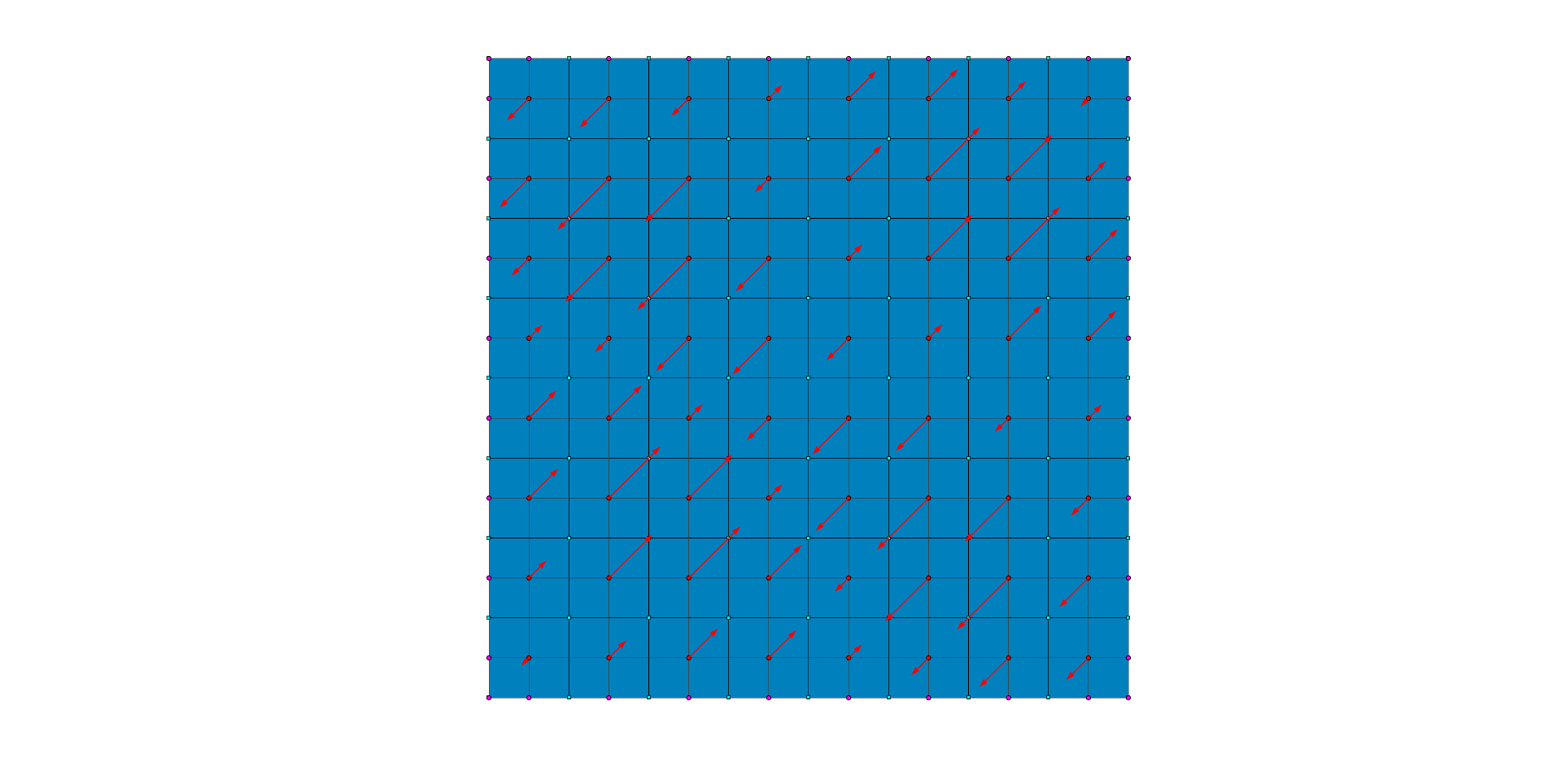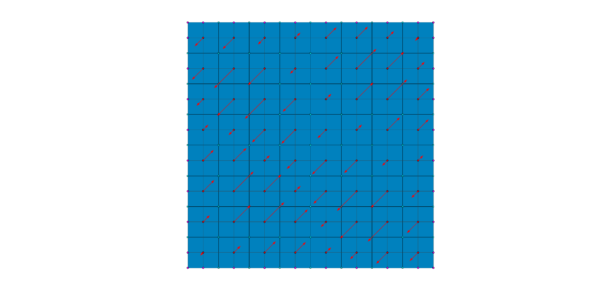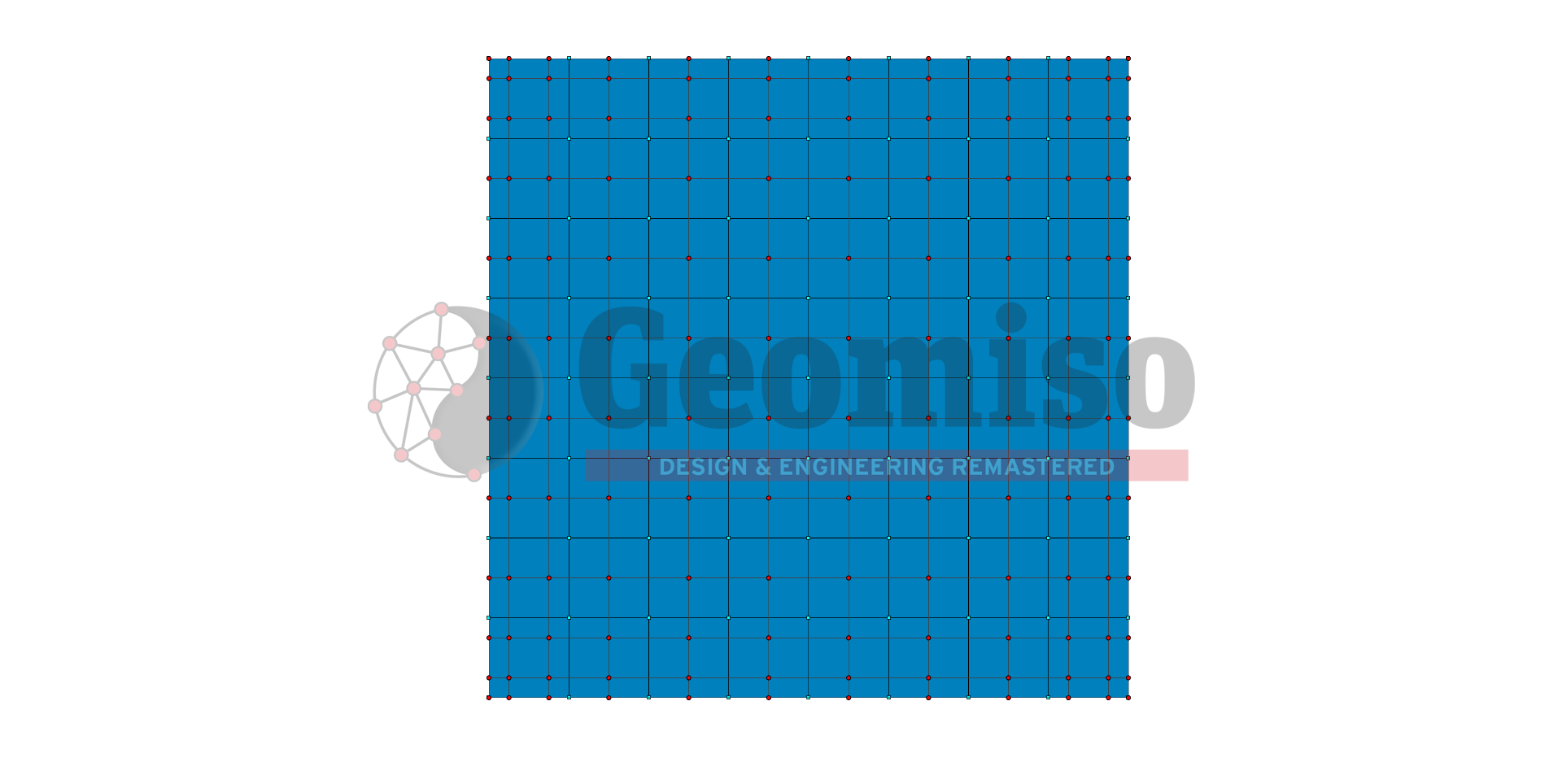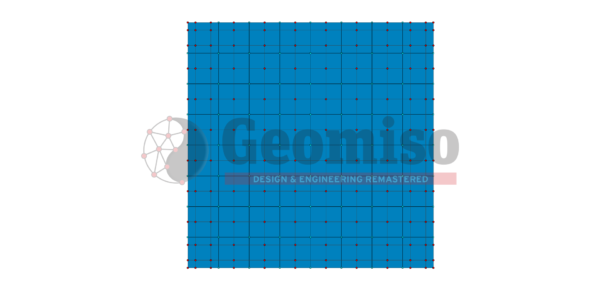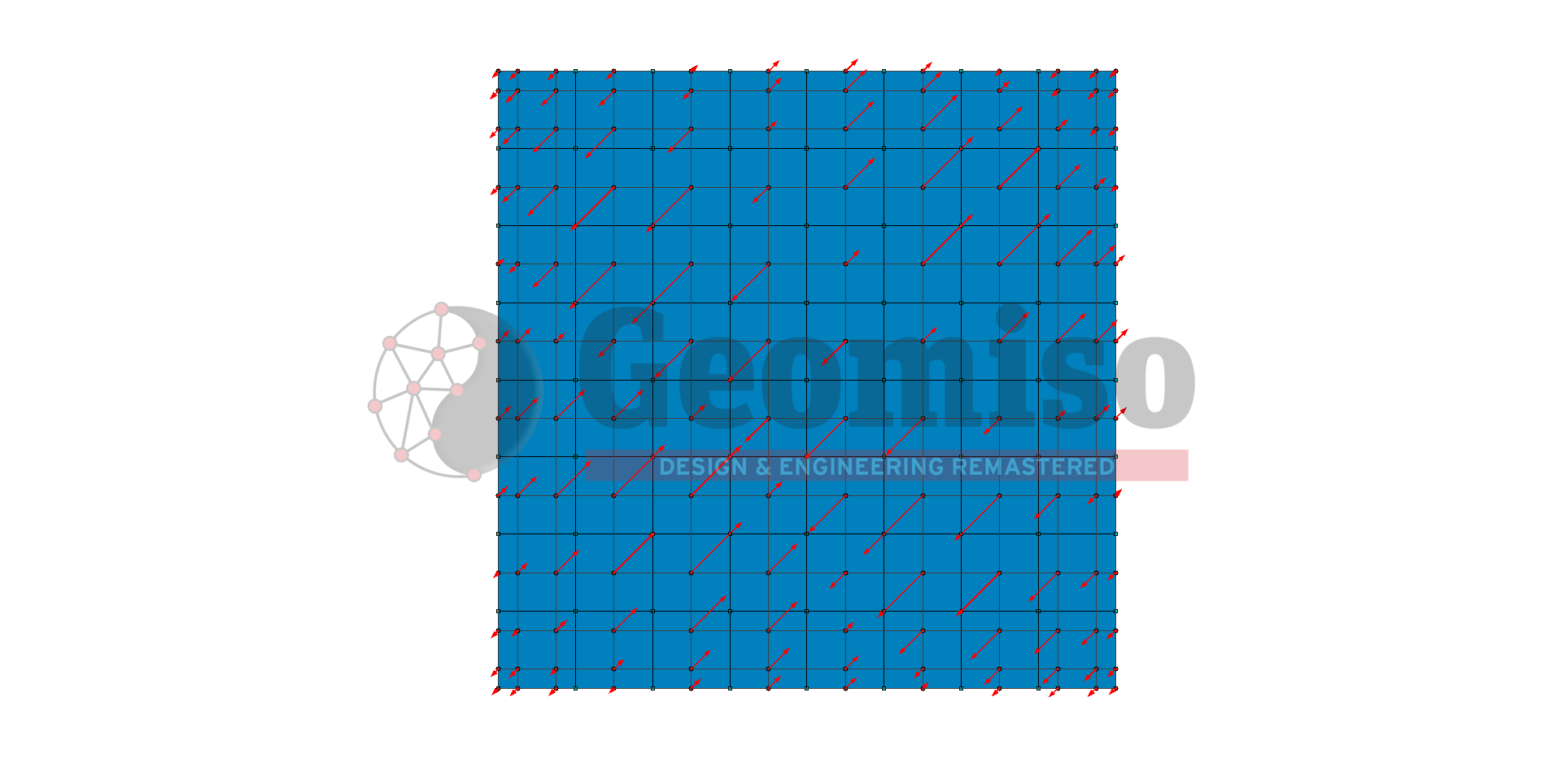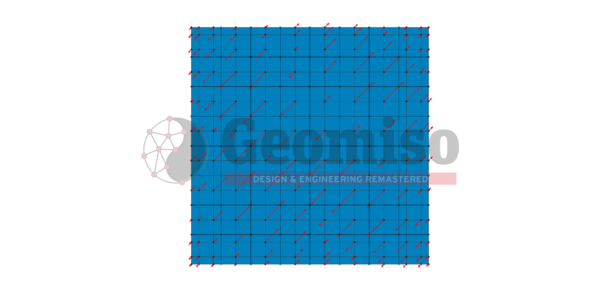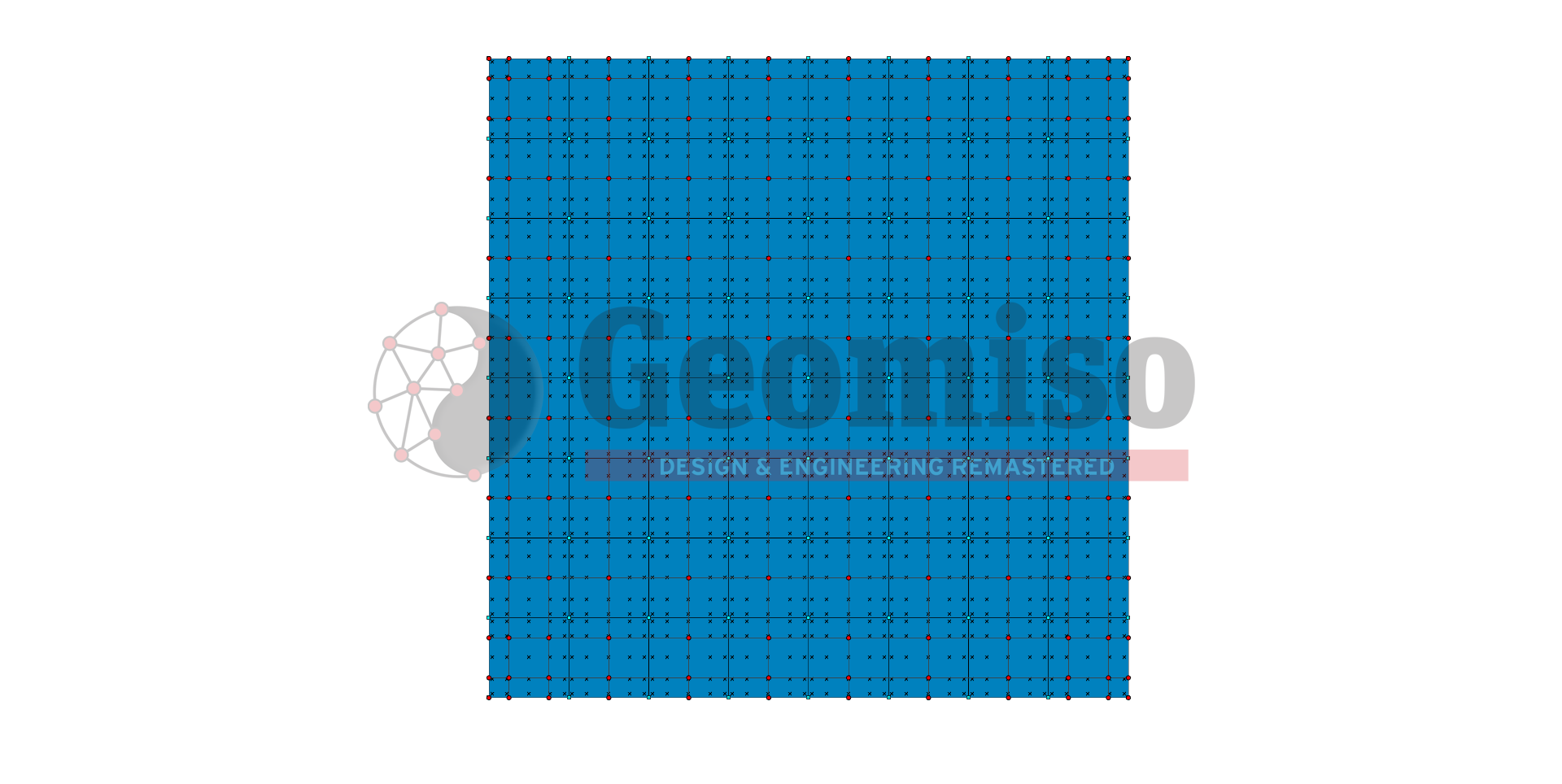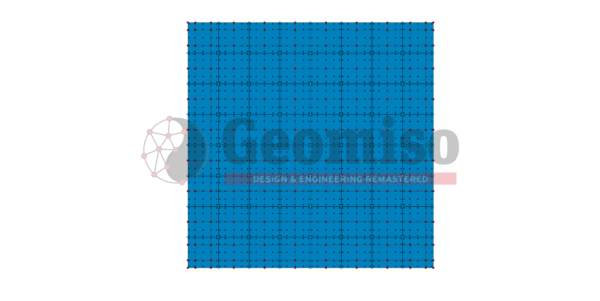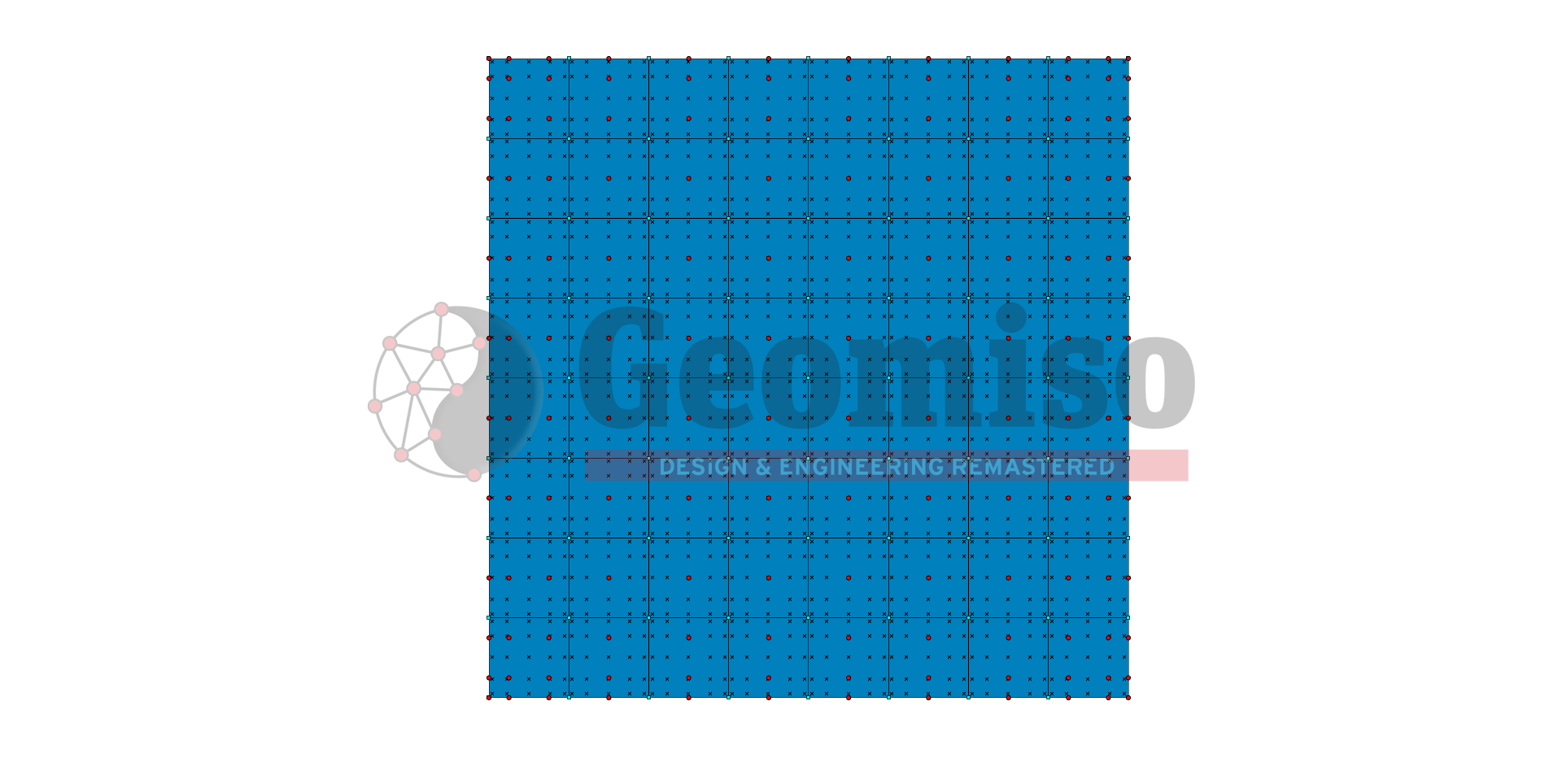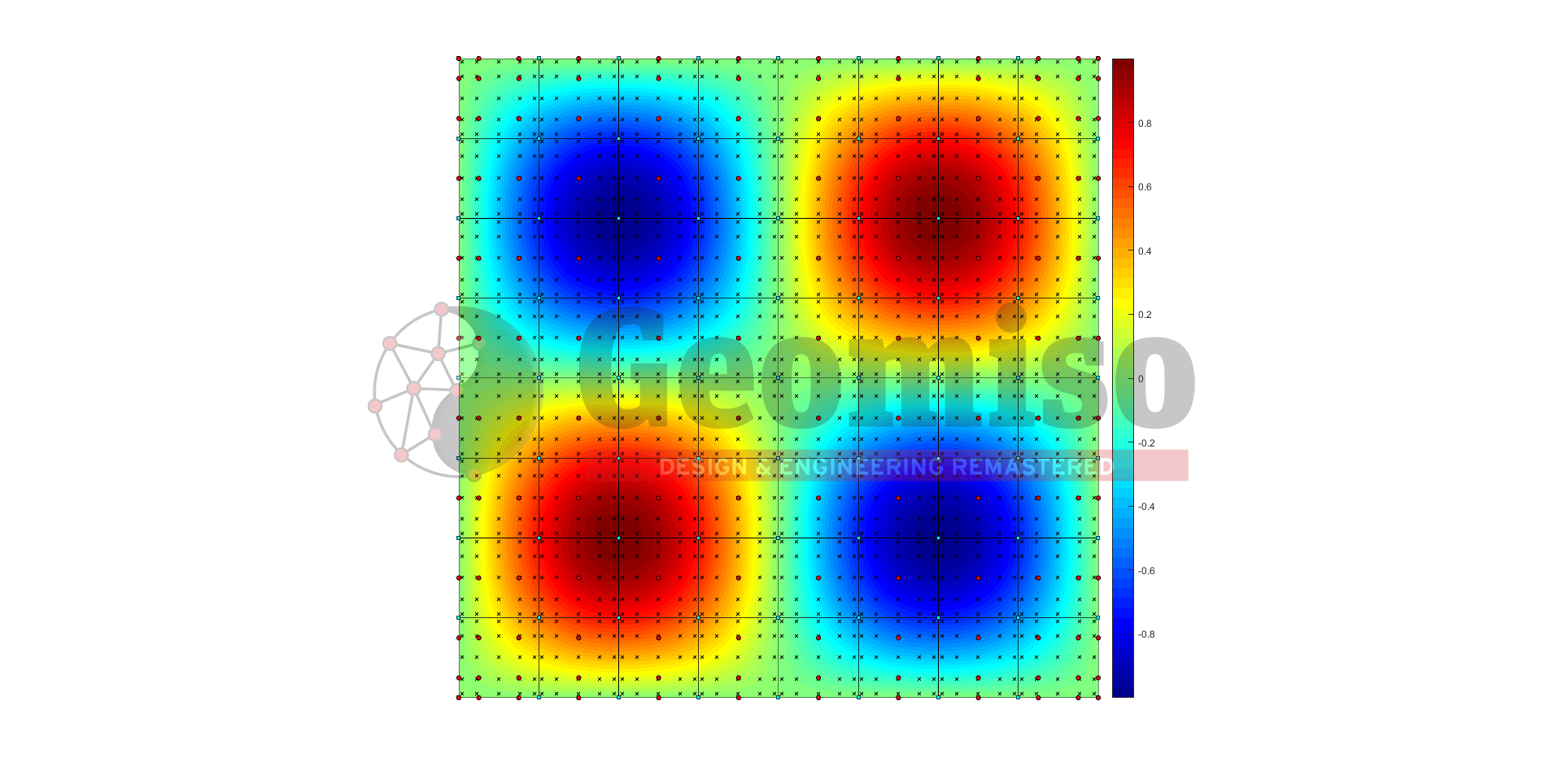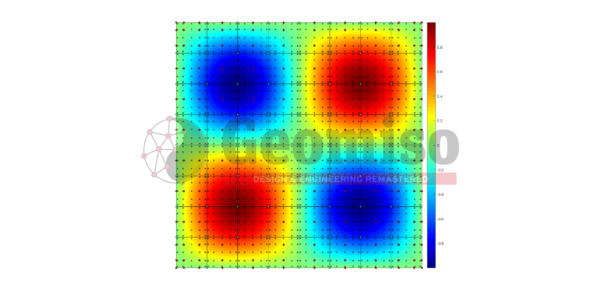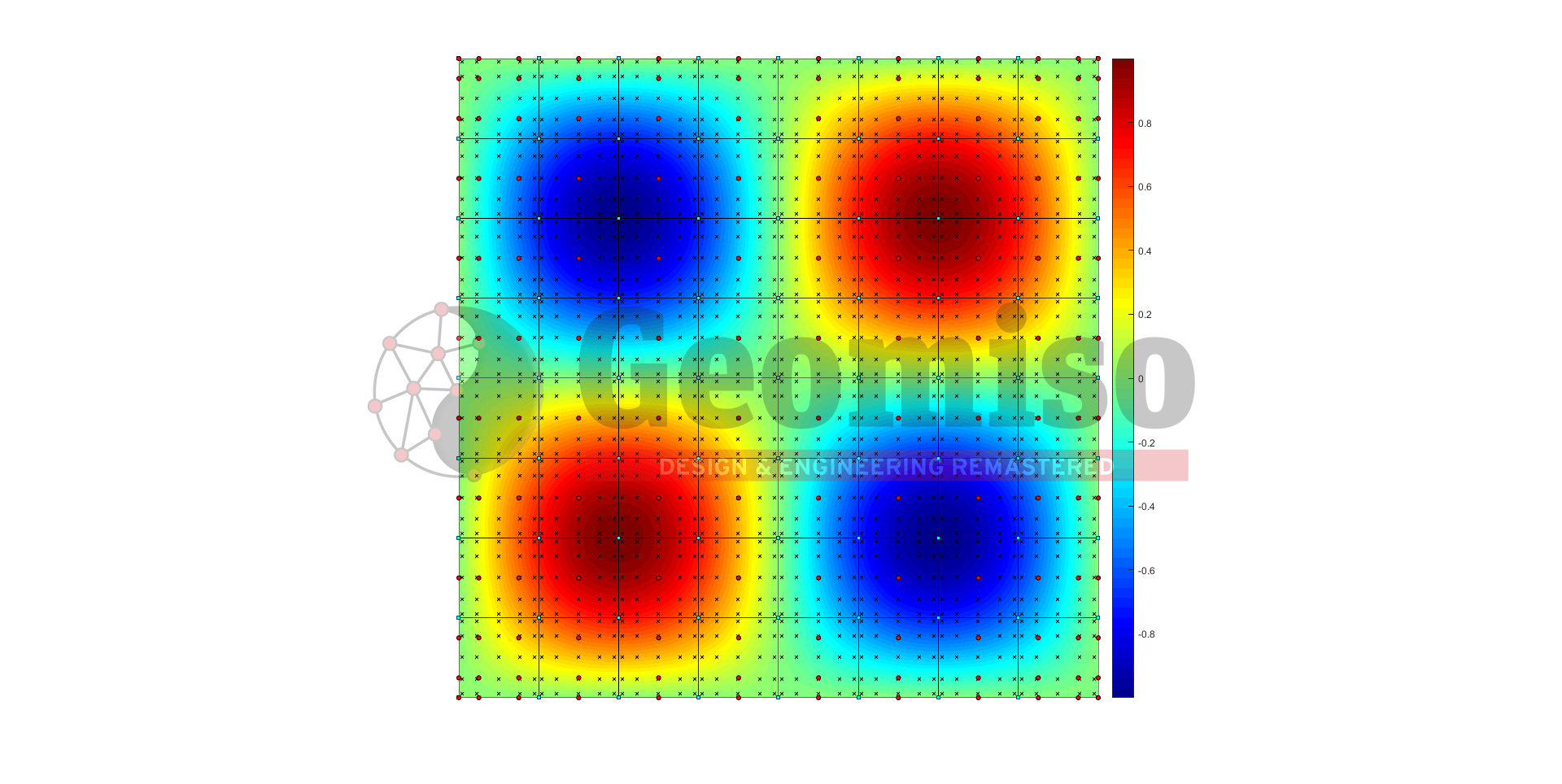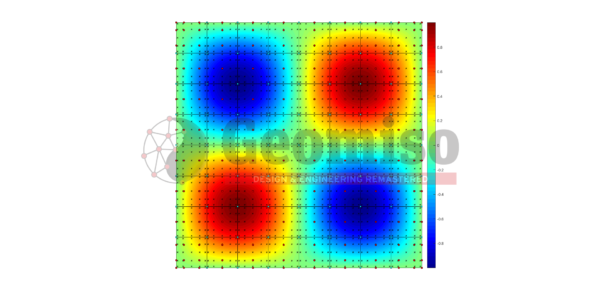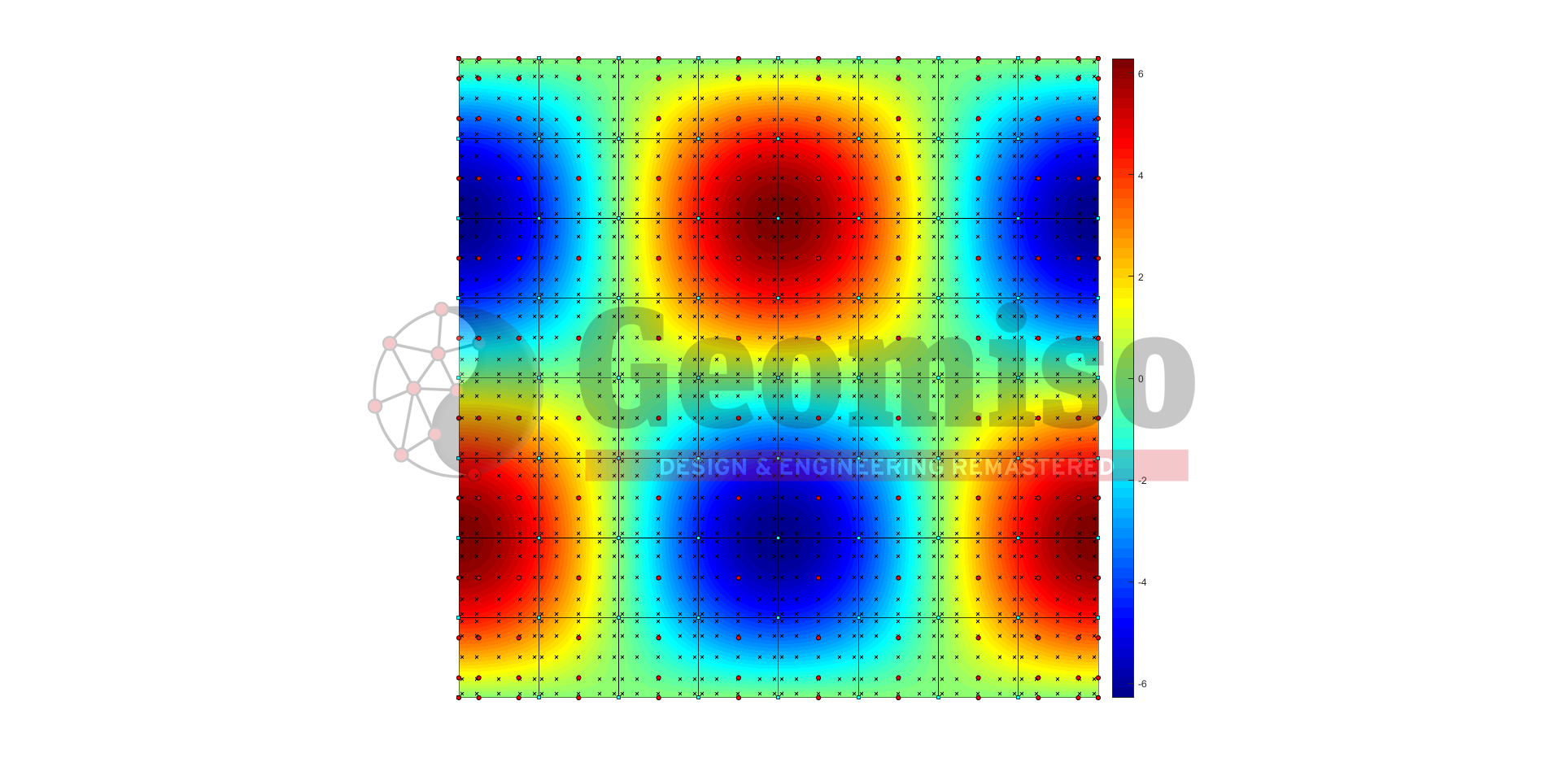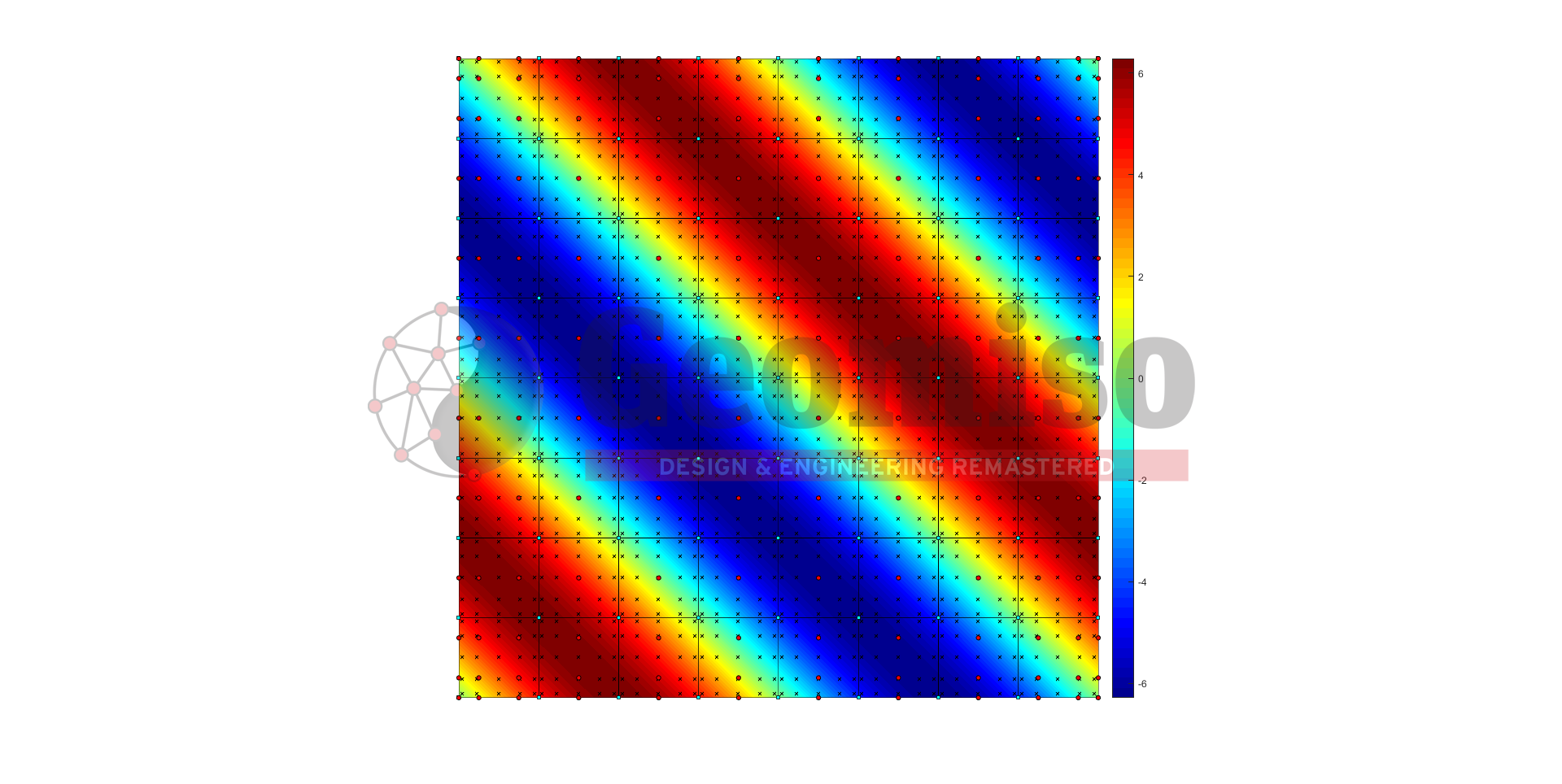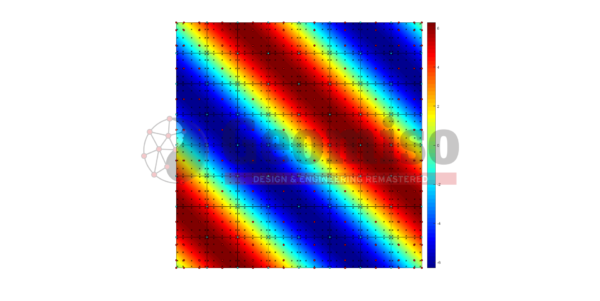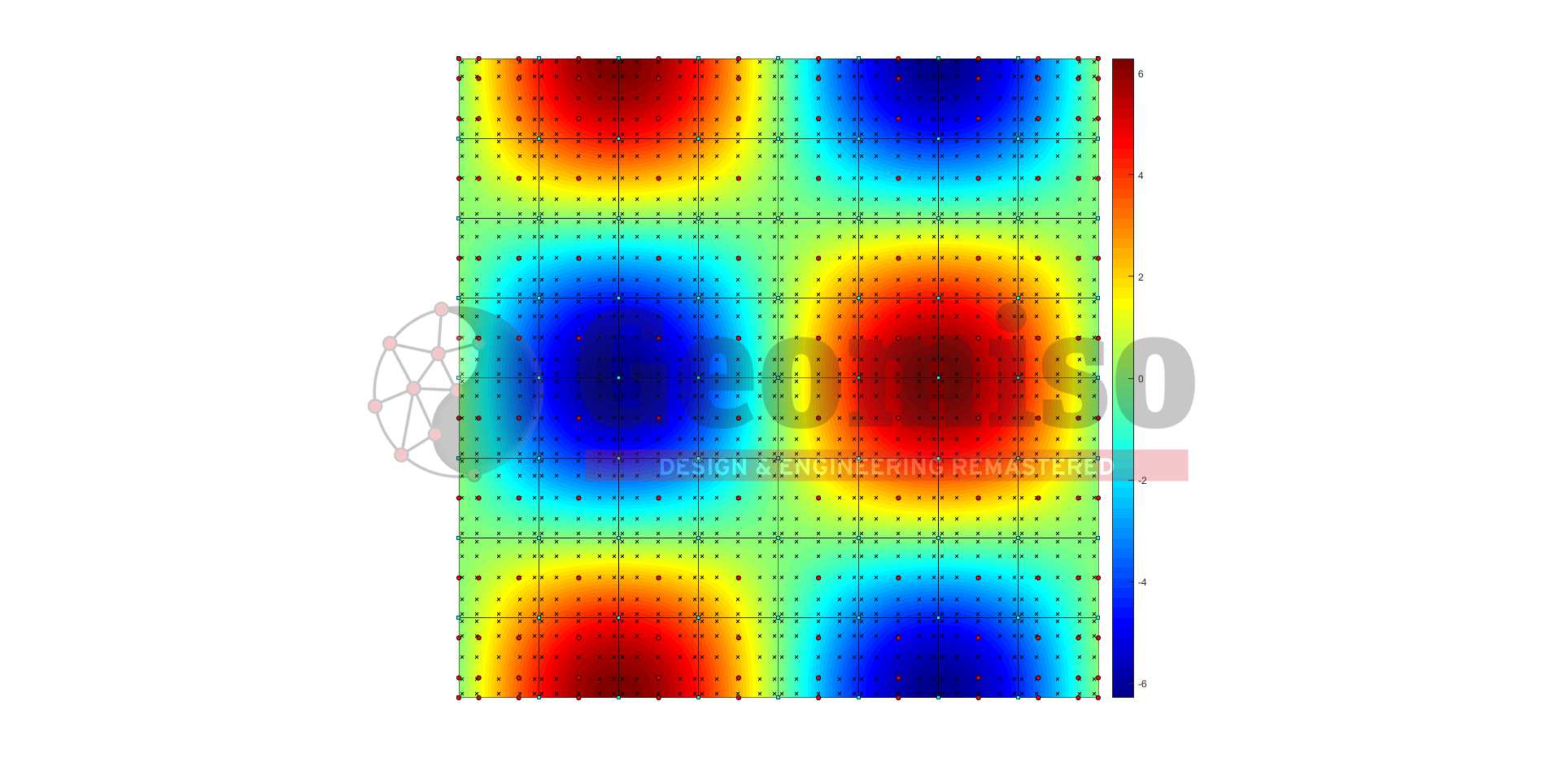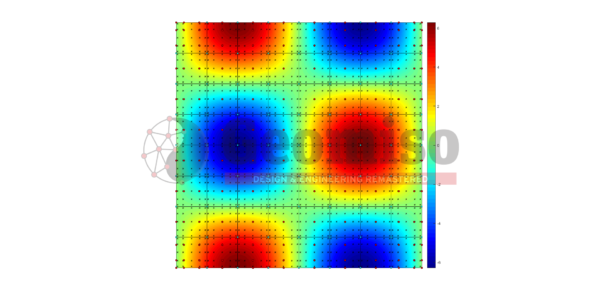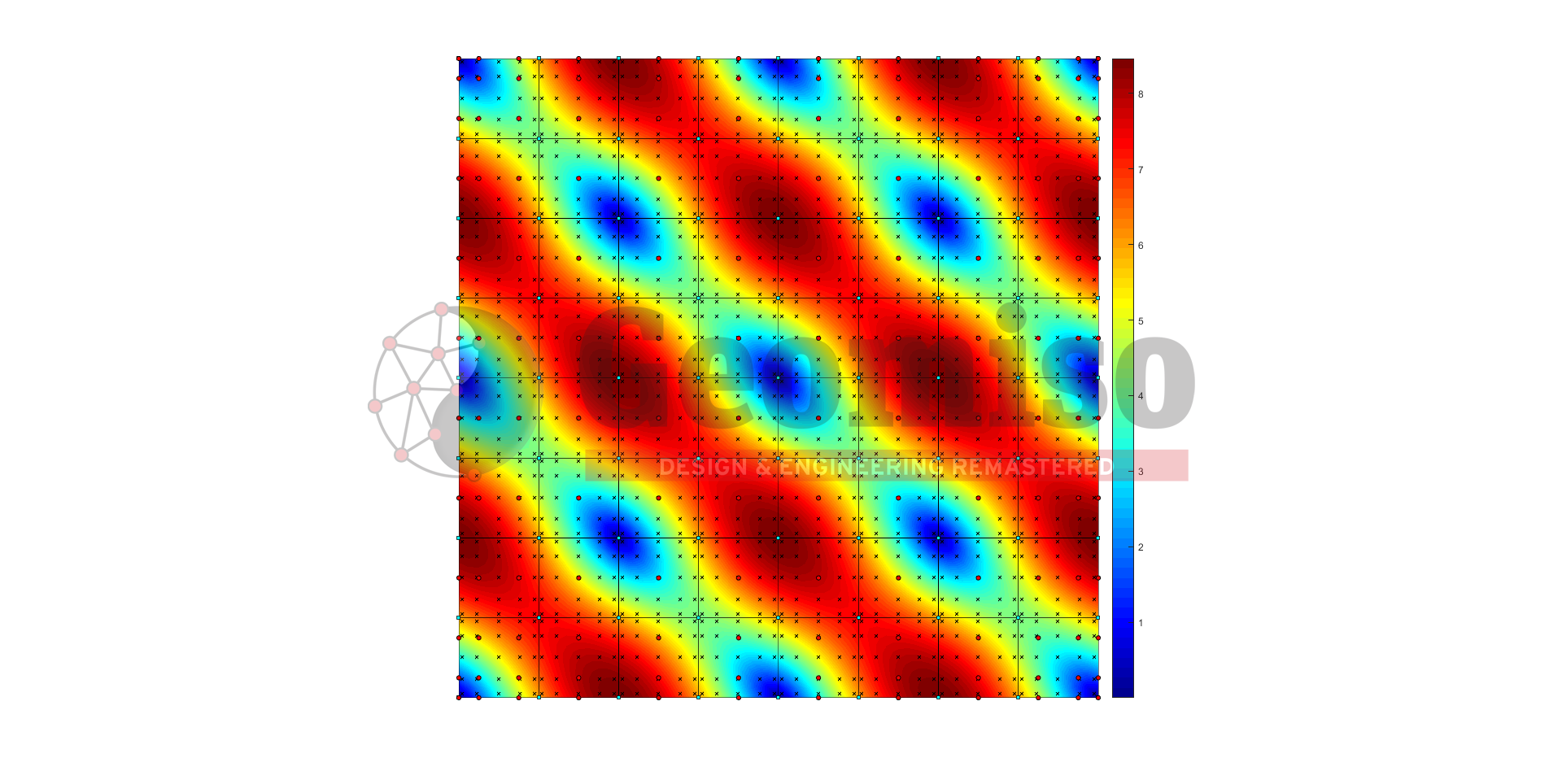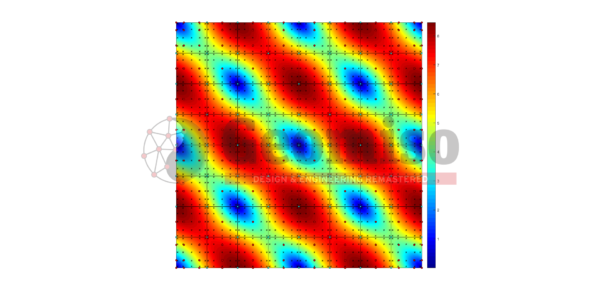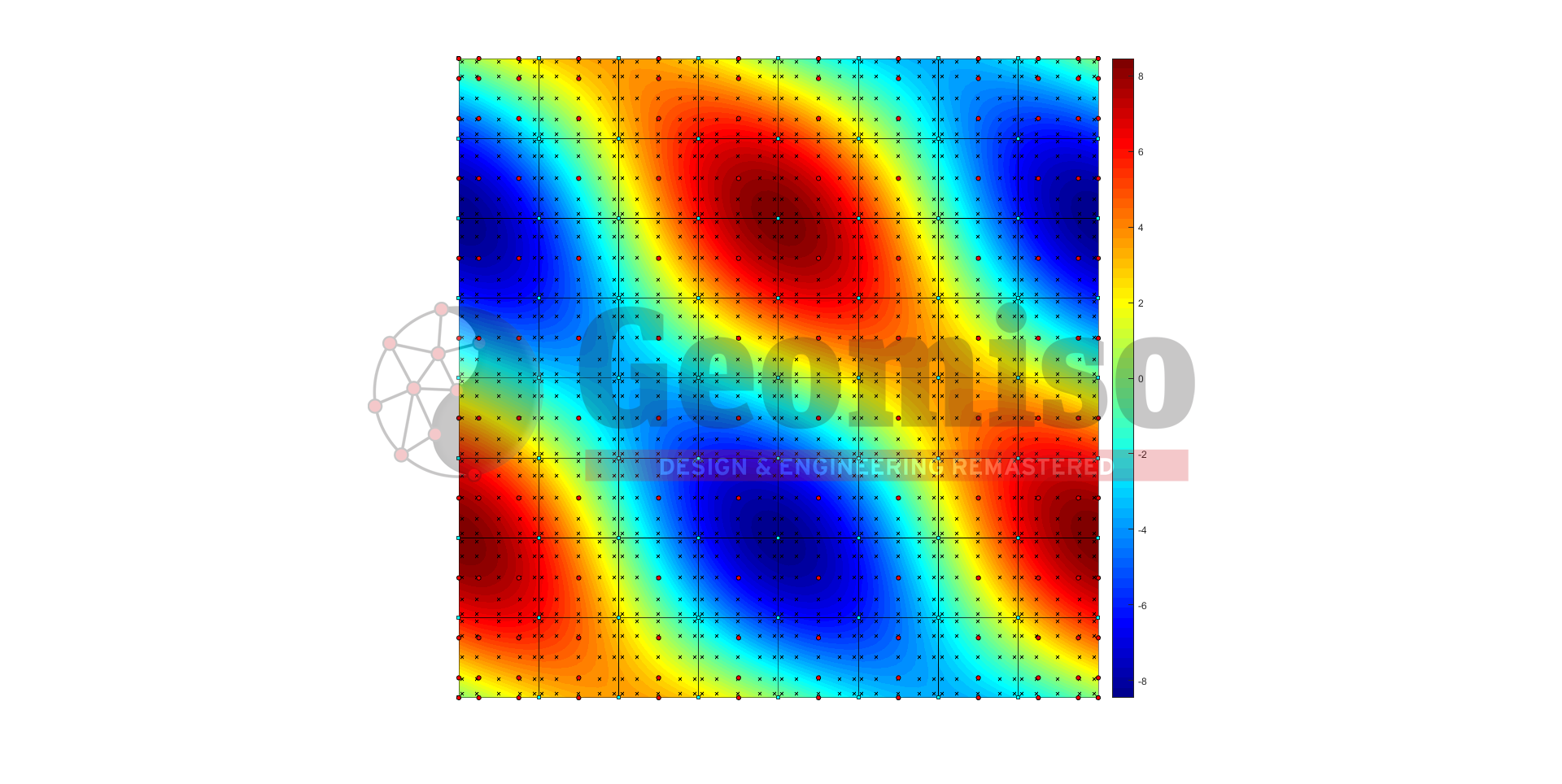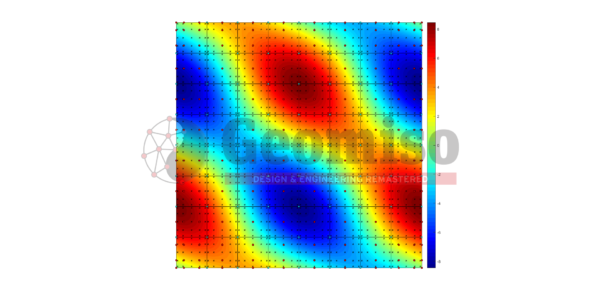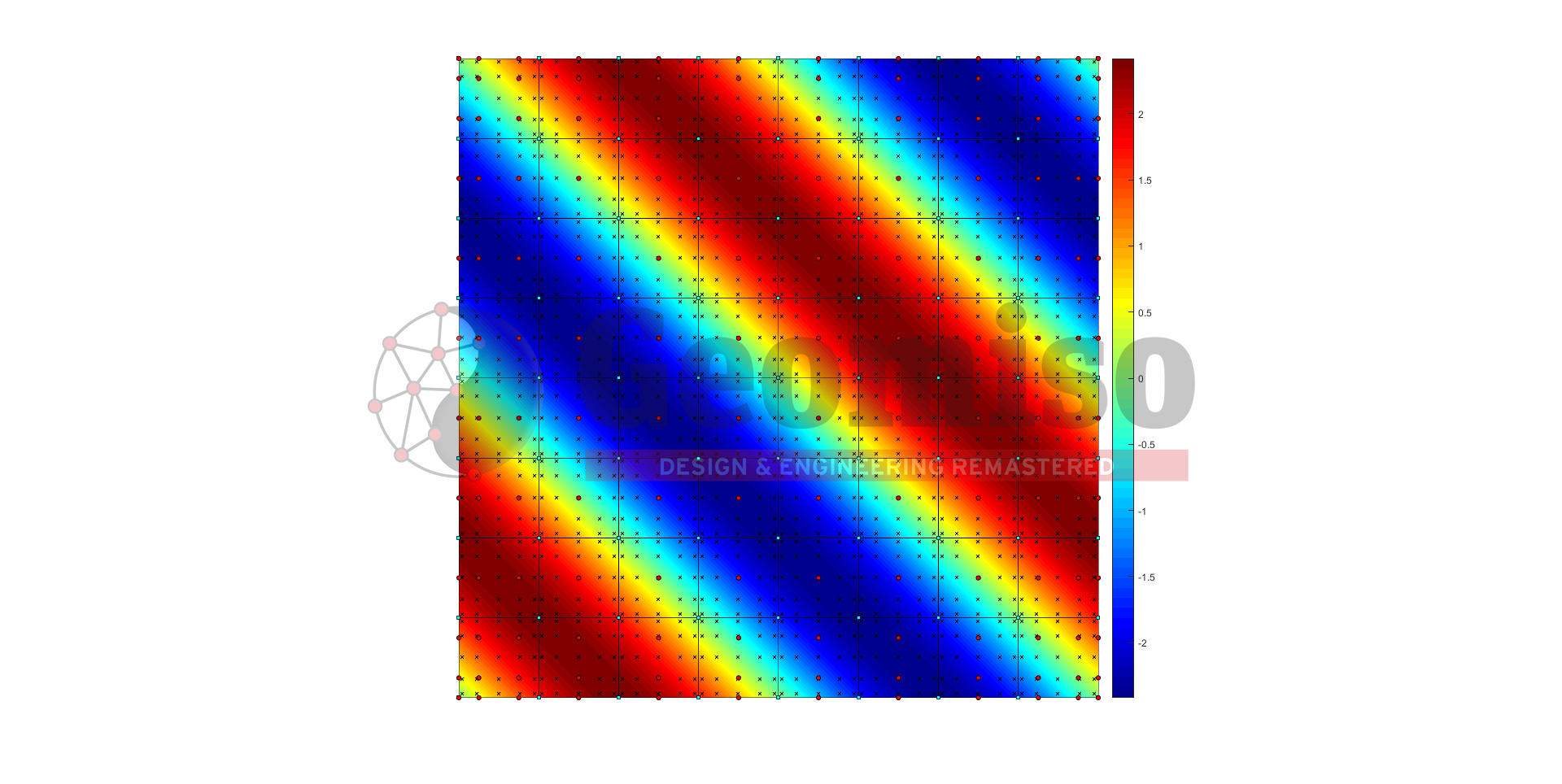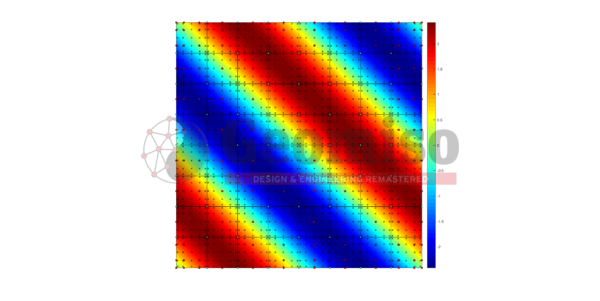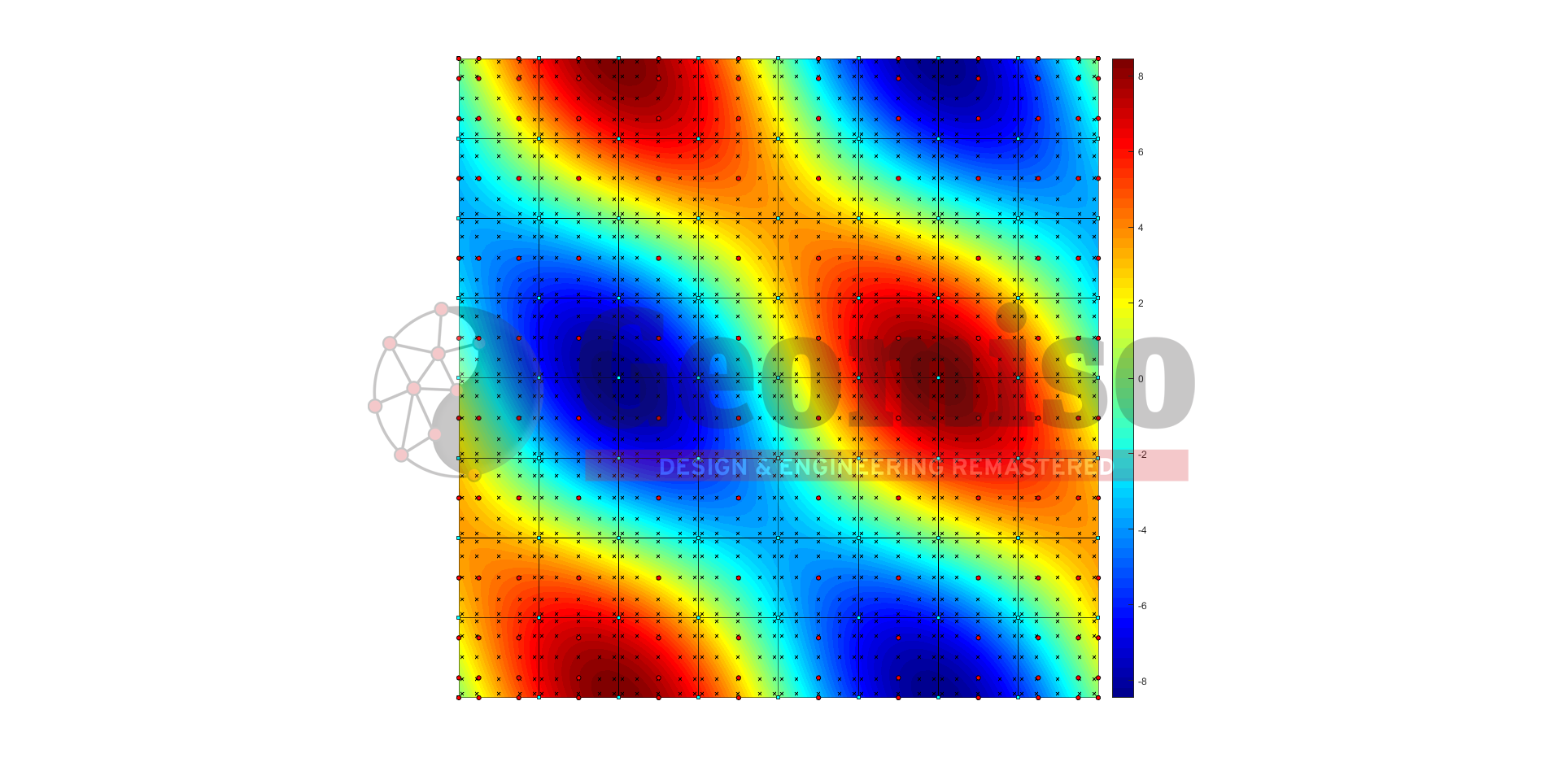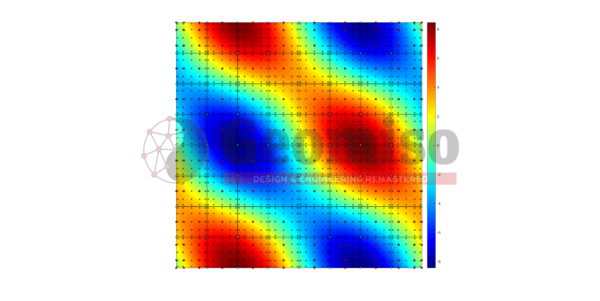# 120001 Poisson’s PDE square v1.0

149,99

This project presents the effect of both knot span number and basis polynomial order on the numerical solution of a Poisson’s PDE with homogeneous Dirichlet boundary conditions, defined in a square domain described with NURBS and subjected to volume loading.

Κατηγορίες: ,

## Περιγραφή

This project presents the effect of both knot span number and basis polynomial order on the numerical solution of a Poisson’s PDE with homogeneous Dirichlet boundary conditions, defined in a square domain described with NURBS and subjected to volume loading. This parametric investigation includes a set of 11 comparisons with a total number of 54 meshes submitted to linear elastic static isogeometric NURBS-based analysis using Geomiso Terminator v1.0. Each comparison provides data about the mesh discretization/ sparsity parameters, the minimum/ maximum value of displacement/ strain/ stress field and the respective error.

Specifications
Product ID 120001
Published July 25, 2018
Size 134 MB
Product Type Project
Mesh Number 54
Geometry Square
Material E=1, ν=1
Constraint Homogeneous Dirichlet
Load Volume
Analysis Type Isogeometric (NURBS) – Static – 2D
Material/ Geometry linearity Linear/ Linear
Quadrature Gauss-Legendre

This pack includes all the following files, which contain the control variables and the knot vectors required to produce the 54 NURBS models, the loading and the boundary conditions needed to solve the problem and the respective results of the 54 analyses (tables, charts, contours) obtained using Geomiso Terminator v1.0 software.

## Αξιολογήσεις

Δεν υπάρχει καμία αξιολόγηση ακόμη.